# Information-Theoretic Entropy Approximants

Shannon  introduced the concept of entropy in information theory, with an eye on its applications in communication theory. The general form of informational entropy (Shannon-Jaynes or relative entropy functional) is [2,3,4]:(1)

whereis a-estimate (prior distribution). The quantityis also referred to as the Kullback-Leibler (KL) distance. As a means for least-biased statistical inference in the presence of testable constraints, Jaynes's used the Shannon entropy to propose the principle of maximum entropy , and if the KL-distance is adopted as the objective functional, the variational principle is known as the principle of minimum relative entropy .

Consider a set of distinct nodes inthat are located at(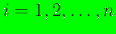), with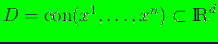denoting the convex hull of the nodal set. For a real-valued function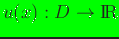, the numerical approximation foris: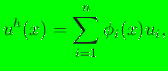(2)

where,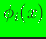is the basis function associated with node, andare coefficients. The use of basis functions that are constructed independent of an underlying mesh has become popular in the past decade--meshfree Galerkin methods are a common target application for such approximation schemes [6,7,8,9,10]. The construction of basis functions using information-theoretic variational principles is a new development [11,12,13,14]; see Reference  for a recent review on meshfree basis functions. To obtain basis functions using the maximum-entropy formalism, the Shannon entropy functional (uniform prior) and a modified entropy functional (Gaussian prior) were introduced in References  and , respectively, which was later generalized by adopting the Shannon-Jaynes entropy functional (any prior) . The implementation of these new basis functions has been carried out, and this manual describes a Fortran 90 library for computing maximum-entropy (max-ent) basis functions and their first and second derivatives for any prior weight function.

We use the relative entropy functional given in Eq. (1) to construct max-ent basis functions. The variational formulation for maximum-entropy approximants is: findas the solution of the following constrained (convex or concave withor, respectively) optimization problem: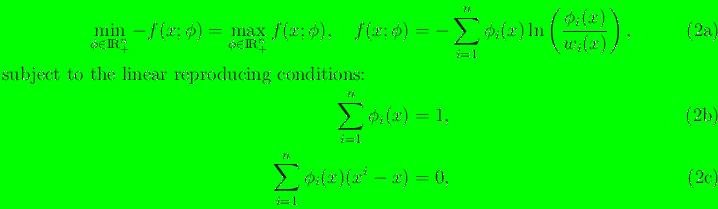whereis the non-negative orthant,is a non-negative weight function (prior estimate to), and the linear constraints form an under-determined system. On using the method of Lagrange multipliers, the solution of the variational problem is :(4)

where() are shifted nodal coordinates,are theLagrange multipliers (implicitly dependent on the point) associated with the constraints in Eq. (3c), andis known as the partition function in statistical mechanics. The smoothness of maximum-entropy basis functions for the Gaussian prior was established in Reference ; the continuity for any() prior was proved in Reference .

N. Sukumar